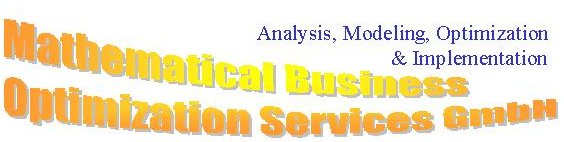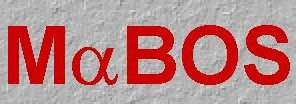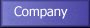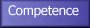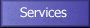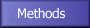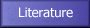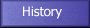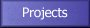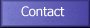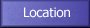MaBOS is specialized in the following optimization methods: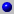LP       (linear programming)MILP   (mixed integer linear programming)NLP    (non-linear programming)MINLP (mixed integer non-linear programmingGLOPT (global optimization)
What is optimization and what are optimization problems? In an optimization problem (OP), one tries to minimize or maximize a global characteristic of a decision process such as elapsed time or cost, by an appropriate choice of degrees of freedom which can be controlled, and under a set of constraints, linked for example to physical limits. Optimization problems arise in almost all branches of industry or society, e.g., in product and process design, production, logistics, traffic control and even strategic planning. Unfortunately, the word optimization, in nontechnical language, is often used in the sense of improving while the original meaning of the word is related to finding the best. A traditional, and certainly not optimal way to develop answers to optimization problems is to propose a number of choices for the decision variables, using heuristic methods and simulation to evaluate the objective function. The processes under investigation are then simulated under these various options, and the results are compared. Experts in charge of these OPs have developed intuition and heuristics to select appropriate conditions, and simulation software exists to perform the evaluation of their performance. These ''traditional'' techniques may lead to proper results, but there is no guarantee that the optimal solution or even a solution close to the optimum is found. This is especially troublesome for complex problems, or those which require decisions with large financial impact. What do we need when we want to solve a real world problem by mathematical optimization? The first thing is we need to represent the real world problem by a mathematical model. A mathematical model of a system is a set of mathematical relationships (e.g., equalities, inequalities, logical conditions) which represent an abstraction of the real world problem under consideration. Usually, a mathematical model in optimization consists of four key objects: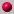datavariables (continuous, semi-continuous, binary, integer),constraints (equalities, inequalities), andobjective function.
The data fix an instance of a problem. They may represent costs or demands, fixed operation conditions of a reactor, capacities of plants and so on. The variables represent the degrees of freedom, i.e., our decision: How much of a certain product is to be produced, whether a depot is closed or not, or how much material will we store in the inventory for later use. The constraints can be a wide range of mathematical relationships: algebraic, differential or integral. They may represent mass balances, quality relations, capacity limits, and so on. The objective function, eventually, expresses our goal: minimize costs, maximize utilization rate, minimize waste and so on. When building mathematical models for optimization they usually lead to structured problems such as:linear programming (LP) problems,mixed integer linear programming (MILP) problems,onlinear programming (NLP) problems, andmixed integer nonlinear programming (MINLP) problems.
Besides building a model and classifying the problem we need a solver, i.e., a piece of software which has a set of algorithms implemented capable of solving the problem listed above. What is discrete (the terms mixed integer optimization and discrete optimization are used synonymously) optimization? Classical optimization theory (calculus, variational calculus, optimal control) treats those cases in which the decision variables can be changed continuously, e.g., the temperature in a chemical reactor or the amount of a product to be produced. On the other hand, mixed integer, combinatorial or discrete optimization addresses degrees of freedom which are limited to integer values, for example counts (numbers of containers, ships), decisions (yes-no), or logical relations (if product A is produced then product B also needs to be produced). This discipline, formerly only a marginal discipline within mathematical optimization, becomes more and more important (Grötschel, 1993) as it extends the power of mathematical optimization to situations which are relevant to practical decision making situations where business success is at stake. What is the difference between simulation and mathematical optimization? In contrast to simulation, mathematical optimization methods search directly for an optimal solution that fulfills all restrictions (constraints) and relations which are relevant to the real-world problem. By using mathematical optimization it becomes possible to control and adjust complex systems even when they are difficult for a human being to grasp. Therefore, optimization techniques allow a fuller exploitation of the advantages inherent to complex systems. There is another very substantial difference between simulation and optimization: the existence of constraints. While in simulation somebody has to make sure that only those combinations of degrees of freedom are evaluated which represent ''appropriate conditions'' in optimization it has to be specified apriori what makes a feasible solution. That leads to the concept of constraints. What commercial potential is in discrete optimization? To give some idea of the scope of mathematical optimization in helping organizations we cite two recent examples of benefits achieved. First, at Delta Airlines it is reported in a paper by Subramanian et al. (1994) that use of an optimization model is expected to save the company \$(US)300 million over a three year period. Secondly, a comprehensive mathematical programming model used by Digital Equipment Corporation (DEC) is described by Arntzen et al (1995). The model has helped the company to save \$(US)100 million. In some blending problems BASF-AG saved several hundred thousands DM/year. Mathematical optimization is an appropriate approach to support decision processes in many areas. The development of new algorithms in mathematical optimization, software and hardware enables us to solve larger and more complex optimization problems in acceptable times. The chemical, refinery and food industry benefits greatly from this progress since many multi-component flow problems due to the presence of the pooling problem lead to nonlinear or even mixed integer nonlinear models. The pooling problem refers to the intrinsic nonlinear problem of forcing the same (unknown) fractional composition of multi-component streams emerging from a pool, e.g., a tank or a splitter in a petrochemical network. Nonlinear nonconvex problems can be solved to global optimality combining convex underestimators with branch-and-bound techniques.

If you have any questions to MaBOS GmbH please send us an e-mail to info@mabos.com.

If there are any network problems please mail the problem to the local Webmaster of MaBOS.